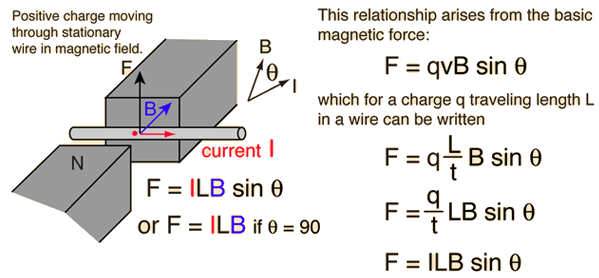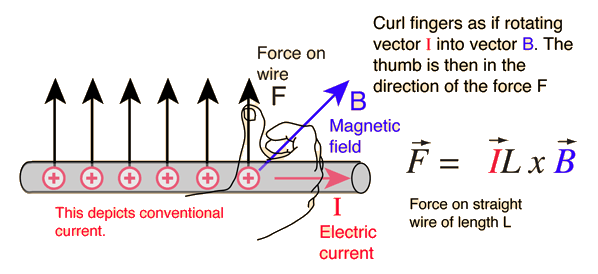# Magnetic Force on a Current-Carrying WireThe magnetic force on a current-carrying wire is perpendicular to both the wire and the magnetic field with direction given by the right hand rule.If the current is perpendicular to the magnetic field then the force is given by the simple product:

## Force = Current x Length x B-field

A current carrying conductor placed in a magnetic field experiences a force. If the direction of the field and that of current are mutually perpendicular to each other, then the force acting on the conductor will be perpendicular to both and that can be determined using the Fleming’s left-hand rule. When current establishes in the conductor, it gets displaced which verifies the existence of a force on the conductor.Fig. (1) –  A current-carrying rod, AB, experiences a force perpendicular to its length and the magnetic field

Source: NCERT class X lab manual

## Fleming’s Left Hand Rule:Fig. (2) –  Fleming’s left hand rule.

Source: NCERT class X lab manual

Hold the thumb and the first two fingers of your left hand mutually at right angles to each other as shown in Fig. (2). Then if the Forefinger points in the direction of the Field, and the second finger in the direction of the Current, the thumb will point in the direction of Force.

Factors affecting magnetic force on a current-carrying conductor in a magnetic field:

• Strength of the magnetic field
• Current flowing through the wire
• Length of the wire

F=BIlsinθ, where

• F is force acting on a current carrying conductor,B is magnetic flux density (magnetic field strength),
• I is magnitude of current flowing through the conductor,
• l
l

is length of conductor,

• θ
θ

is angle that conductor makes with the magnetic field.

When the conductor is perpendicular to the magnetic field, the force will be maximum. When it is parallel to the magnetic field, the force will be zero.

## STEM Elearning

We at FAWE have built this platform to aid learners, trainers and mentors get practical help with content, an interactive platform and tools to power their teaching and learning of STEM subjects, more

#### How to find your voice as a woman in Africa

© FAWE, Powered by: Yaaka DN.